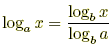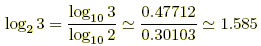index: click on a letter A B C D E F G H I J K L M N O P Q R S T U V W X Y Z A to Z index index: subject areas numbers & symbols sets, logic, proofs geometry algebra trigonometry advanced algebra & pre-calculus calculus advanced topics probability & statistics real world applications multimedia entrieswww.mathwords.com about mathwords website feedback

Change of Base Formula

A formula that allows you to rewrite a logarithm in terms of logs written with another base. This is especially helpful when using a calculator to evaluate a log to any base other than 10 or e.

 Assume that x, a, and b are all positive. Also assume that a ≠ 1, b ≠ 1. Change of base formula:Example 1:Example 2:(note that)   Example 3: